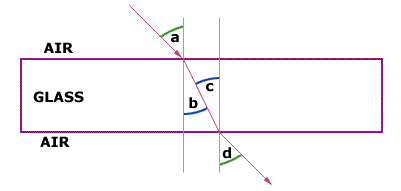# Physics LIGHT AND SOUND PROBLEMSPanda1) If Angle "a" is 25o, Angle "b" is:2) If Angle "a" is 25o, Angle "b" is:3) If Angle "a" is 25o, Angle "d" is:

4) f the wavelength of orange light is 6 · 10-7 m, calculate its frequency.

f =

6) For a closer look in shaving, a:

7) Full-length mirrors used while dressing are:

8) A 2.0 X 2.0 -inch slide is placed before a converging lens 21 inch in front of the focal point. If the focal length is 20. inches, how large is the image that is projected on the screen?

11) Four beats per second are heard when an unknown tuning fork and a 240 Hz tuning fork are struck simultaneously. What are the two possible frequencies of the tuning fork?

12) The echo of your footsteps returns in 0.50 second. How long is the corridor in which you are walking? (Assume the speed of sound for 0oC.)

[removed] m

• Posted: 7 years ago
• Due:
• Budget: \$20